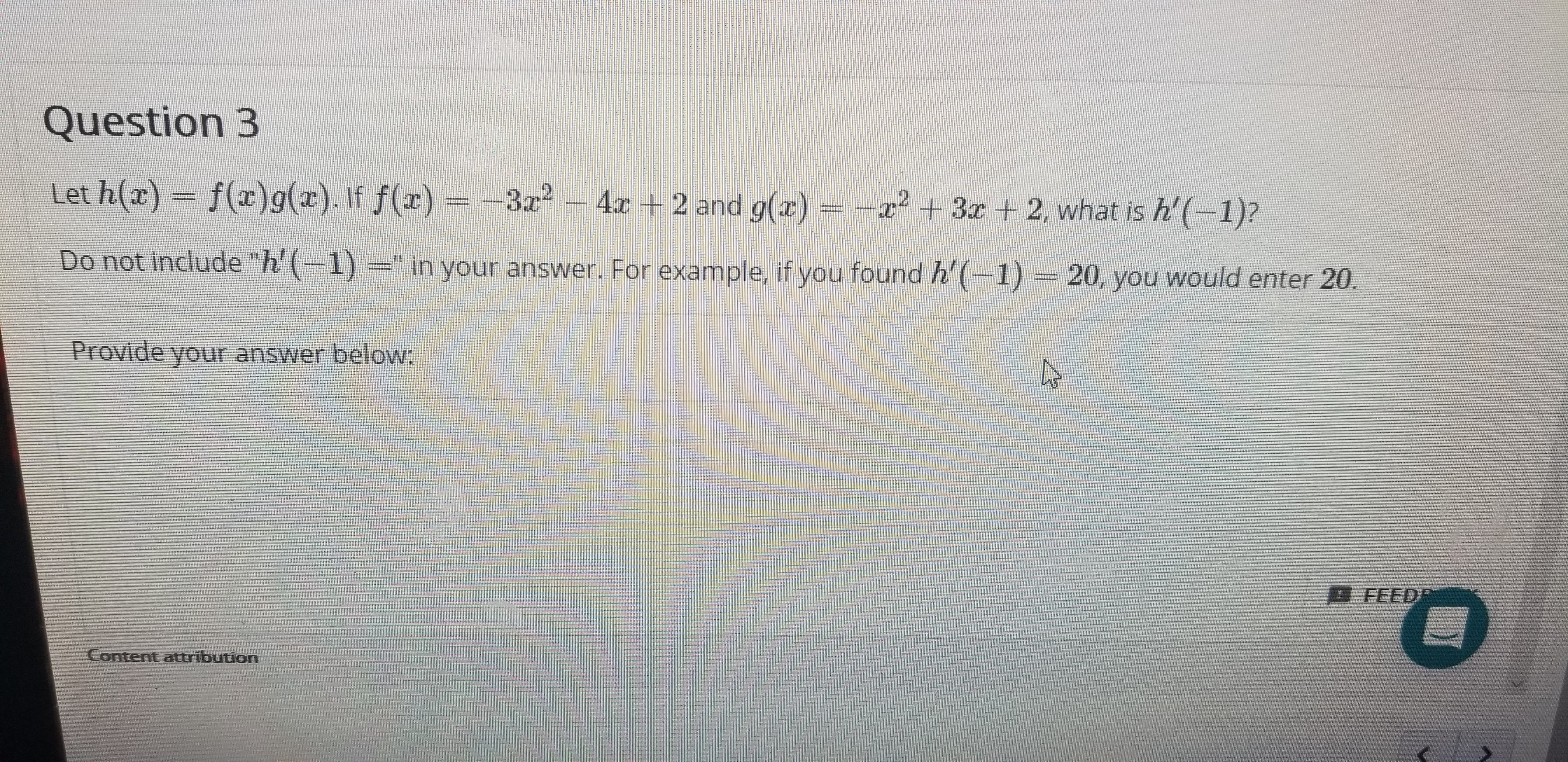# Question 3 Let h(x) f(x)g(a). If f(x) -3a2 -4x 2 and g(x) = -x +3x +2 , what is h'(-1)? Do not include "h'(--1) =" in your answer. For example, if you found h'(-1) = 20, you would enter 20. Provide your answer below: FEED Content attribution

Questionhelp_outlineImage TranscriptioncloseQuestion 3 Let h(x) f(x)g(a). If f(x) -3a2 -4x 2 and g(x) = -x +3x +2 , what is h'(-1)? Do not include "h'(--1) =" in your answer. For example, if you found h'(-1) = 20, you would enter 20. Provide your answer below: FEED Content attribution fullscreen

### Want to see this answer and more?

Experts are waiting 24/7 to provide step-by-step solutions in as fast as 30 minutes!*

*Response times vary by subject and question complexity. Median response time is 34 minutes and may be longer for new subjects.
Tagged in
MathCalculus

### Other